Exponent shift method for power series summation

Description of the method

This method is used for summing up various power series that look similar to existing power series but have some terms missing, extra, or shifted. It is closely related to another method, the substitution method for power series summation.

Missing terms in summation

Suppose we know how to do a certain summation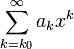$\sum_{k=k_0}^\infty a_kx^k$, and we are asked to do a summation$\sum_{k=k_1}^\infty a_kx^k$, where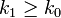$k_1 \ge k_0$ is a positive integer. This is given by: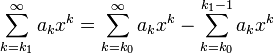$\sum_{k = k_1}^\infty a_kx^k = \sum_{k=k_0}^\infty a_kx^k - \sum_{k=k_0}^{k_1 - 1} a_kx^k$

Shifted index of summation

Suppose we know how to do a summation of the form:$\sum_{k=k_0}^\infty a_kx^k$

Let's now consider another summation, with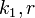$k_1, r$ integers such that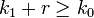$k_1 + r \ge k_0$:$\sum_{k=k_1}^\infty a_{k+r}x^k$

We simplify this as follows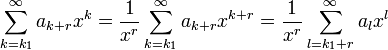$\sum_{k=k_1}^\infty a_{k+r}x^k = \frac{1}{x^r}\sum_{k = k_1}^\infty a_{k + r}x^{k + r} = \frac{1}{x^r} \sum_{l = k_1 + r}^\infty a_lx^l$

We now use the missing terms in summation idea to simplify this final summation in terms of the original.

Examples

Series we need to sum Type Rewrite in terms of known series Final answer$\sum_{k=1}^\infty \frac{x^k}{k!}$ Missing terms only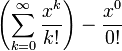$\left(\sum_{k=0}^\infty \frac{x^k}{k!} \right) - \frac{x^0}{0!}$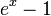$e^x - 1$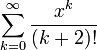$\sum_{k=0}^\infty \frac{x^k}{(k + 2)!}$ Shifted index of summation + missing terms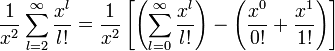$\frac{1}{x^2}\sum_{l=2}^\infty \frac{x^l}{l!} = \frac{1}{x^2} \left[\left(\sum_{l=0}^\infty \frac{x^l}{l!}\right) - \left(\frac{x^0}{0!} + \frac{x^1}{1!}\right)\right]$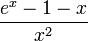$\frac{e^x - 1 - x}{x^2}$, expression valid for$x \ne 0$. At$x = 0$, we get a value of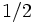$1/2$.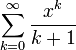$\sum_{k=0}^\infty \frac{x^k}{k + 1}$ Shifted index of summation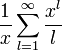$\frac{1}{x} \sum_{l=1}^\infty \frac{x^l}{l}$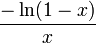$\frac{-\ln(1 - x)}{x}$ for$x \in [-1,0) \cup (0,1)$, 1 for$x = 0$.